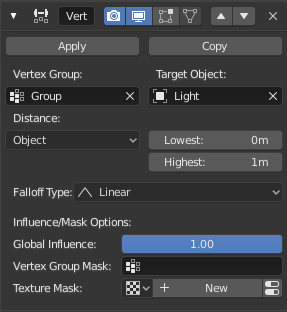# Vertex Weight Proximity Modifier¶

This modifier sets the weights of the given vertex group, based on the distance between the object (or its vertices), and another target object (or its geometry).

Warning

This modifier does implicit clamping of weight values in the standard (0.0 to 1.0) range. All values below 0.0 will be set to 0.0, and all values above 1.0 will be set to 1.0.

Note

You can view the modified weights in Weight Paint Mode. This also implies that you will have to disable the Vertex Weight Proximity modifier if you want to see the original weights of the vertex group you are editing.

## Options¶The Vertex Weight Proximity modifier panel.

Vertex Group
The vertex group to affect.
Target Object
The object from which to compute distances.
Proximity mode
Object Distance
Use the distance between the modified mesh object and the target object as weight for all vertices in the affected vertex group.
Geometry Distance

Use the distance between each vertex and the target object, or its geometry.

Vertex
This will set each vertex’s weight from its distance to the nearest vertex of the target object.
Edge
This will set each vertex’s weight from its distance to the nearest edge of the target object.
Face
This will set each vertex’s weight from its distance to the nearest face of the target object.

Note

If you enable more than one of them, the shortest distance will be used. If the target object has no geometry (e.g. an empty or camera), it will use the location of the object itself.

Lowest
Distance mapping to 0.0 weight.
Highest
Distance mapping to 1.0 weight.

Tip

Lowest can be set above Highest to reverse the mapping.

Falloff Type

Type of mapping:

Linear
No mapping.
Custom Curve
Allows the user to manually define the mapping using a curve.
Sharp, Smooth, Root and Sphere
These are classical mapping functions, from spikiest to roundest.
Random
Uses a random value for each vertex.
Median Step
Creates binary weights (0.0 or 1.0), with 0.5 as cutting value.